# Data Visualization in R

Data visualization is the technique used to deliver insights in data using visual cues such as graphs, charts, maps, and many others. This is useful as it helps in intuitive and easy understanding of the large quantities of data and thereby make better decisions regarding it.

The popular data visualization tools that are available are Tableau, Plotly, R, Google Charts, Infogram, and Kibana. The various data visualization platforms have different capabilities, functionality and use cases. They also require a different skill set. This article discusses the use of R for data visualization.

R is a language that is designed for statistical computing, graphical data analysis, and scientific research. It is usually preferred for data visualization as it offers flexibility and minimum required coding through its packages.

Consider the following airquality data set for visualization in R.

Ozone Solar R. Wind Temp Month Day
41 190 7.4 67 5 1
36 118 8.0 72 5 2
12 149 12.6 74 5 3
18 313 11.5 62 5 4
NA NA 14.3 56 5 5
28 NA 14.9 66 5 6

### Types of Data Visualizations

Some of the various types of visualizations offered by R are:

#### Bar Plot

There are two types of bar plots- horizontal and vertical which represent data points as horizontal or vertical bars of certain lengths proportional to value of the data item. They are generally used for continuous and categorical variable plotting. By setting the horiz parameter to true and false, we can get horizontal and vertical bar plots respectively.

Example 1:

 `# Horizontal Bar Plot for  ` `# Ozone concentration in air ` `barplot(airquality\$Ozone, main ``=` `'Ozone Concenteration in air'``, ` `        ``xlab ``=` `'ozone levels'``, horiz ``=` `TRUE) `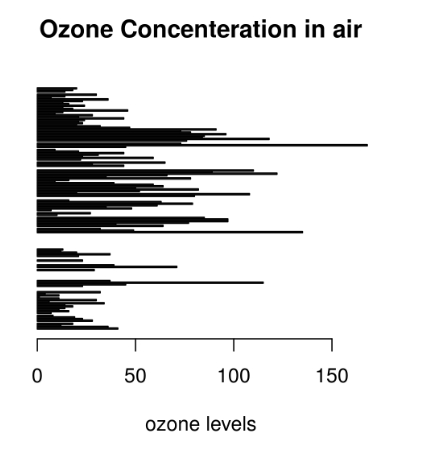Example 2:

 `# Vertical Bar Plot for  ` `# Ozone concentration in air ` `barplot(airquality\$Ozone, main ``=` `'Ozone Concenteration in air'``,  ` `        ``xlab ``=` `'ozone levels'``, col ``=``'blue'``, horiz ``=` `FALSE) `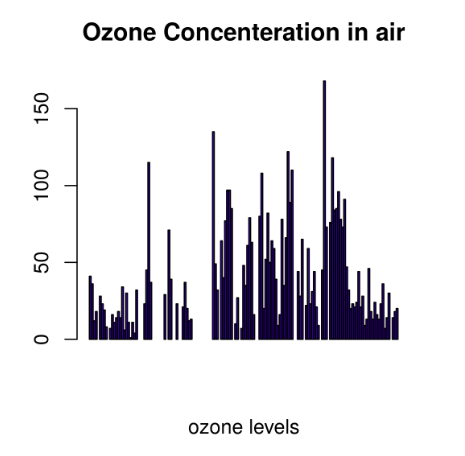Bar plots are used for the following scenarios:

• To perform a comparative study between the various data categories in the data set.
• To analyze the change of a variable over time in months or years.

#### Histogram

A histogram is like a bar chart as it uses bars of varying height to represent data distribution. However, in a histogram values are grouped into consecutive intervals called bins. In a Histogram, continuous values are grouped and displayed in these bins whose size can be varied.

Example:

 `# Histogram for Maximum Daily Temperature ` `data(airquality) ` ` `  `hist(airquality\$Temp, main ``=``"La Guardia Airport's Maximum Temperature(Daily)"``,  ` `     ``xlab ``=``"Temperature(Fahrenheit)"``,  ` `     ``xlim ``=` `c(``50``, ``125``), col ``=``"yellow"``,  ` `     ``freq ``=` `TRUE) `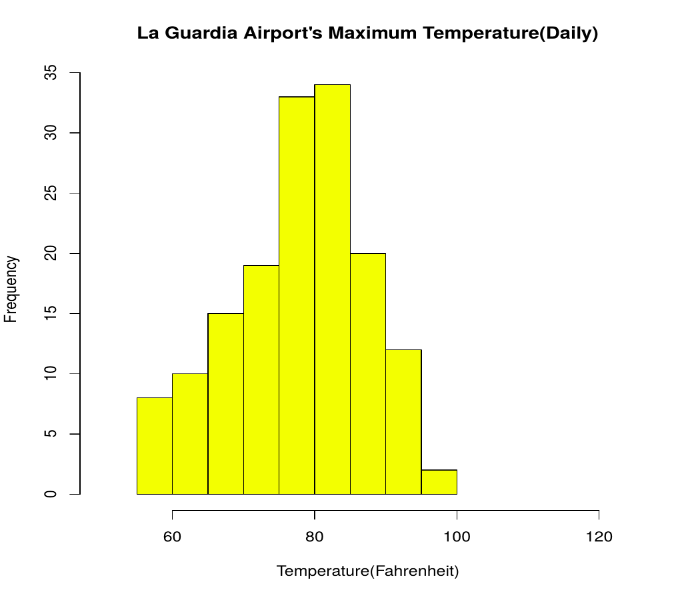For a histogram, the parameter xlim can be used to specify the interval within which all values are to be displayed.
Another parameter freq when set to TRUE denotes the frequency of the various values in the histogram and when set to FALSE, the probability densities are represented on the y-axis such that ther are of the histogram adds up to one.

Histograms are used in the following scenarios:

To verify an equal and symmetric distribution of the data.
• To identify deviations from expected values.

#### Box Plot

Statistical summary of the given data is presented graphically using a boxplot. A boxplot depicts information like the minimum and maximum data point, the median value, first and third quartile and interquartile range.

Example:

 `# Box plot for average wind speed ` `data(airquality) ` ` `  `boxplot(airquality\$Wind, main ``=` `"Average wind speed at La Guardia Airport"``, ` `        ``xlab ``=` `"Miles per hour"``, ylab ``=` `"Wind"``,  ` `        ``col ``=` `"orange"``, border ``=` `"brown"``,  ` `        ``horizontal ``=` `TRUE, notch ``=` `TRUE) `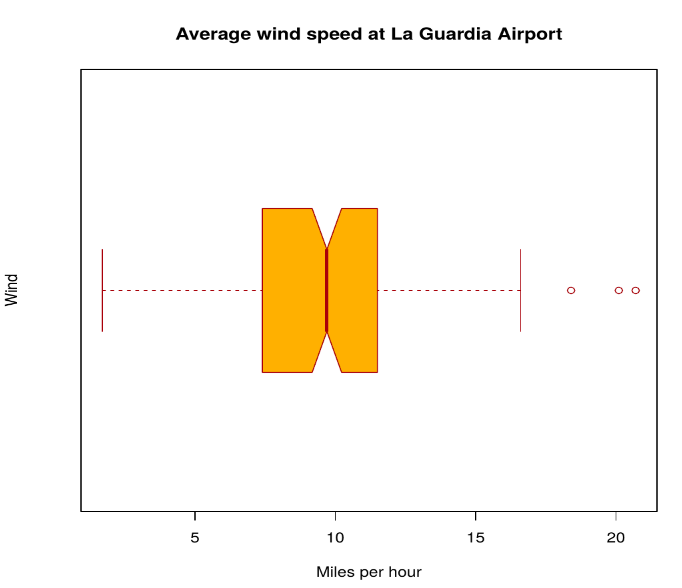Multiple box plots can also be generated at once through the following code:

Example:

 `# Multiple Box plots, each representing ` `# an Air Quality Parameter ` `boxplot(airquality[, ``0``:``4``],  ` `        ``main ``=``'Box Plots for Air Quality Parameters'``) `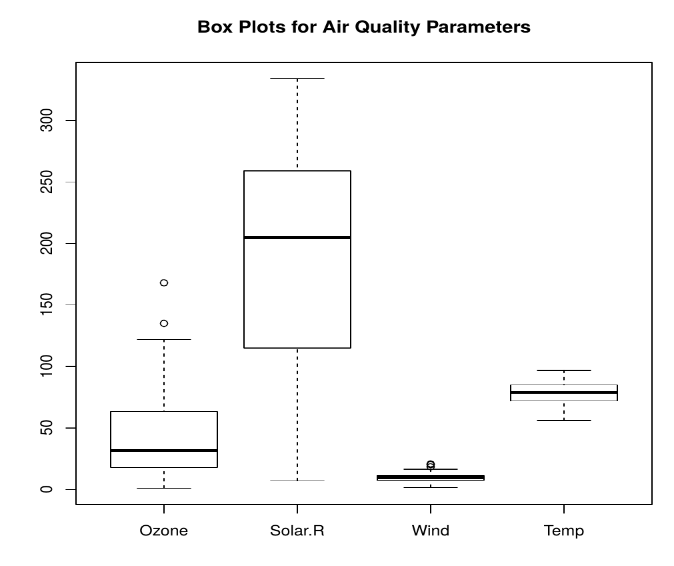Box Plots are used for:

• To give a comprehensive statistical description of the data through a visual cue.
• To identify the outlier points that do not lie in the inter-quartile range of data.

#### Scatter Plot

A scatter plot is composed of many points on a Cartesian plane. Each point denotes the value taken by two parameters and helps us easily identify the relationship between them.

Example:

 `# Scatter plot for Ozone Concentration per month ` `data(airquality) ` ` `  `plot(airquality\$Ozone, airquality\$Month, main ``=``"Scatterplot Example"``, ` `     ``xlab ``=``"Ozone Concentration in parts per billion"``,  ` `     ``ylab ``=``" Month of observation "``, pch ``=` `19``) `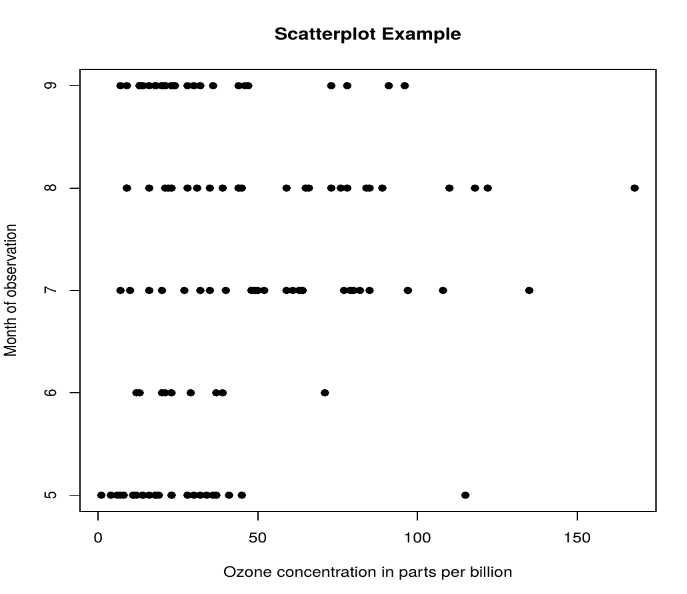Scatter Plots are used in the following scenarios:

• To show whether an association exists between bivariate data.
• To measure the strength and direction of such a relationship.

Advantages of Data Visualization in R: R has the following advantages over other tools for data visualization:

• R offers a broad collection of visualization libraries along with extensive online guidance on their usage.
• R also offers data visualization in the form of 3D models and multipanel charts.
• Through R, we can easily customize our data visualization by changing axes, fonts, legends, annotations, and labels.

• R is only preferred for data visualization when done on an individual standalone server.
• Data visualization using R is slow for large amounts of data as compared to other counterparts.

Application Areas:

• Presenting analytical conclusions of the data to the non-analyst departments of your company.
• Health monitoring devices use data visualization to track any anomaly in blood pressure, cholesterol and others.
• To discover repeating patterns and trends in consumer and marketing data.
• Meteorologists use data visualization for assessing prevalent weather changes throughout the world.
• Real-time maps and geo-positioning systems use visualization for traffic monitoring and estimating travel time.

My Personal Notes arrow_drop_upCheck out this Author's contributed articles.

If you like GeeksforGeeks and would like to contribute, you can also write an article using contribute.geeksforgeeks.org or mail your article to contribute@geeksforgeeks.org. See your article appearing on the GeeksforGeeks main page and help other Geeks.

Please Improve this article if you find anything incorrect by clicking on the "Improve Article" button below.

Article Tags :

Be the First to upvote.

Please write to us at contribute@geeksforgeeks.org to report any issue with the above content.